210405 Epaper

 A Section B SectionC Section D Section210404 Epaper

 A Section B SectionC Section D Section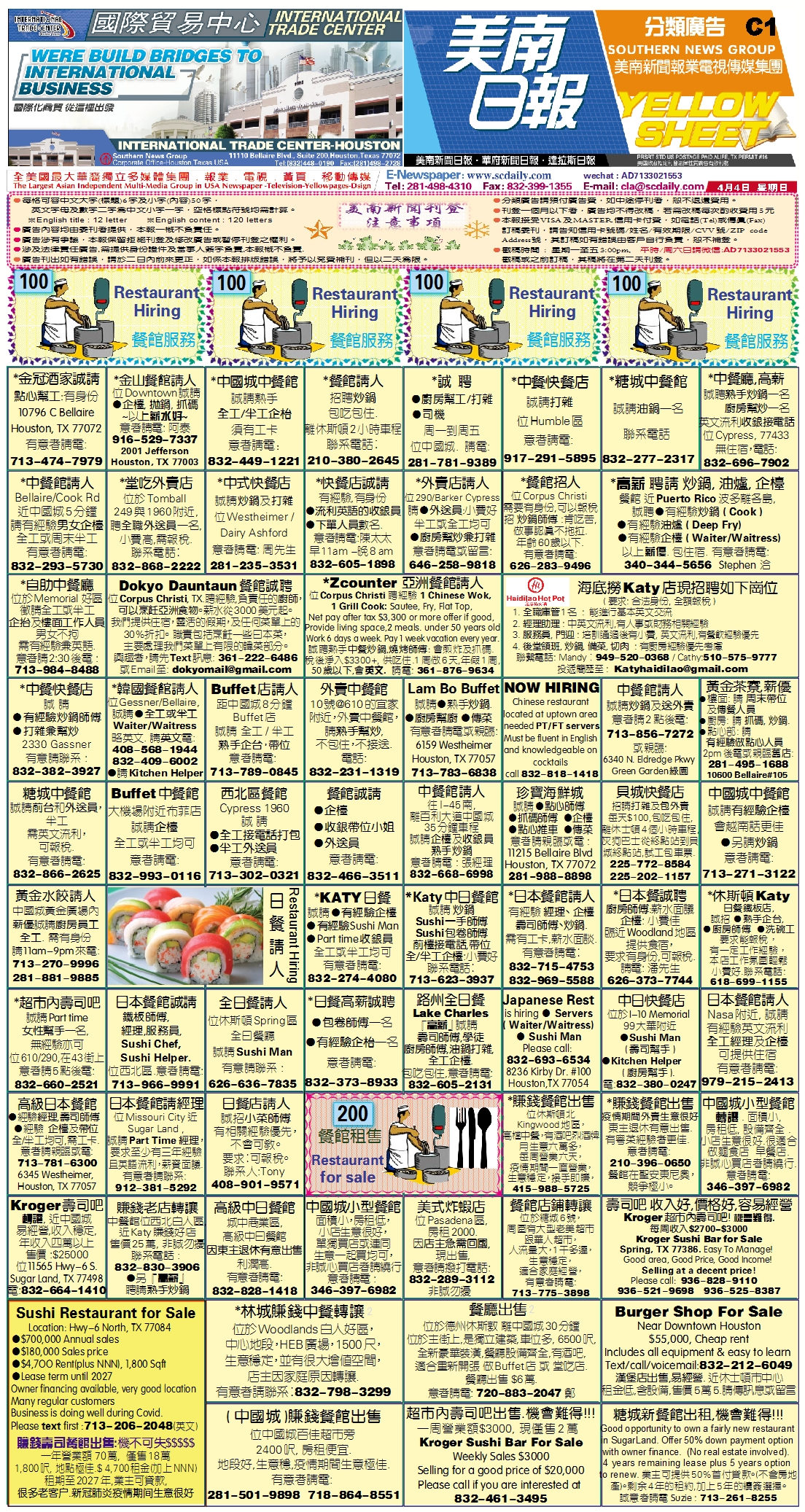210403 Epaper

 A Section B SectionC Section D Section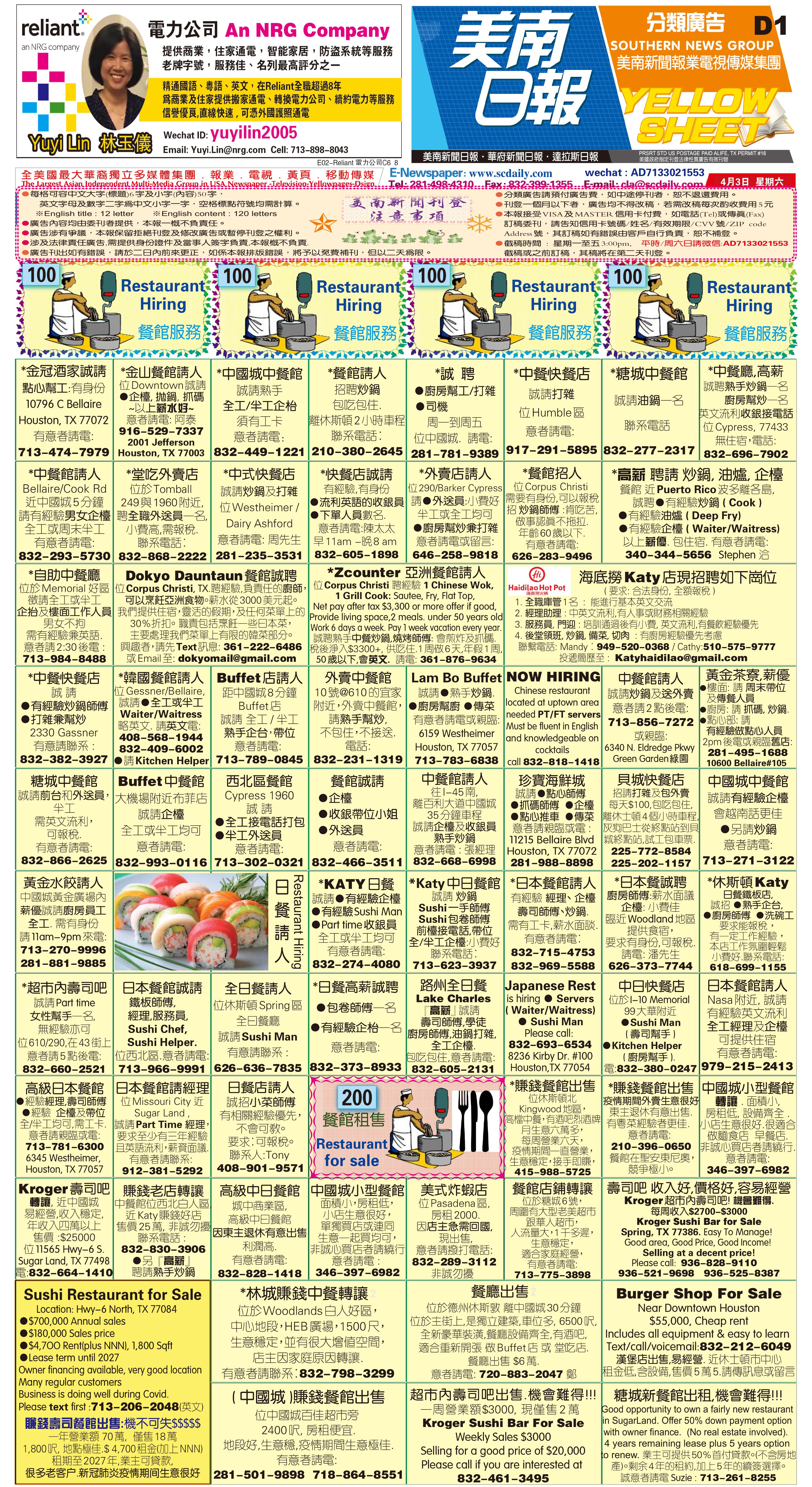E Section (English)>  >

210402 Epaper

 A Section B SectionC Section D SectionE Section (English)>  >

210401 Epaper

 A Section B SectionC Section D Section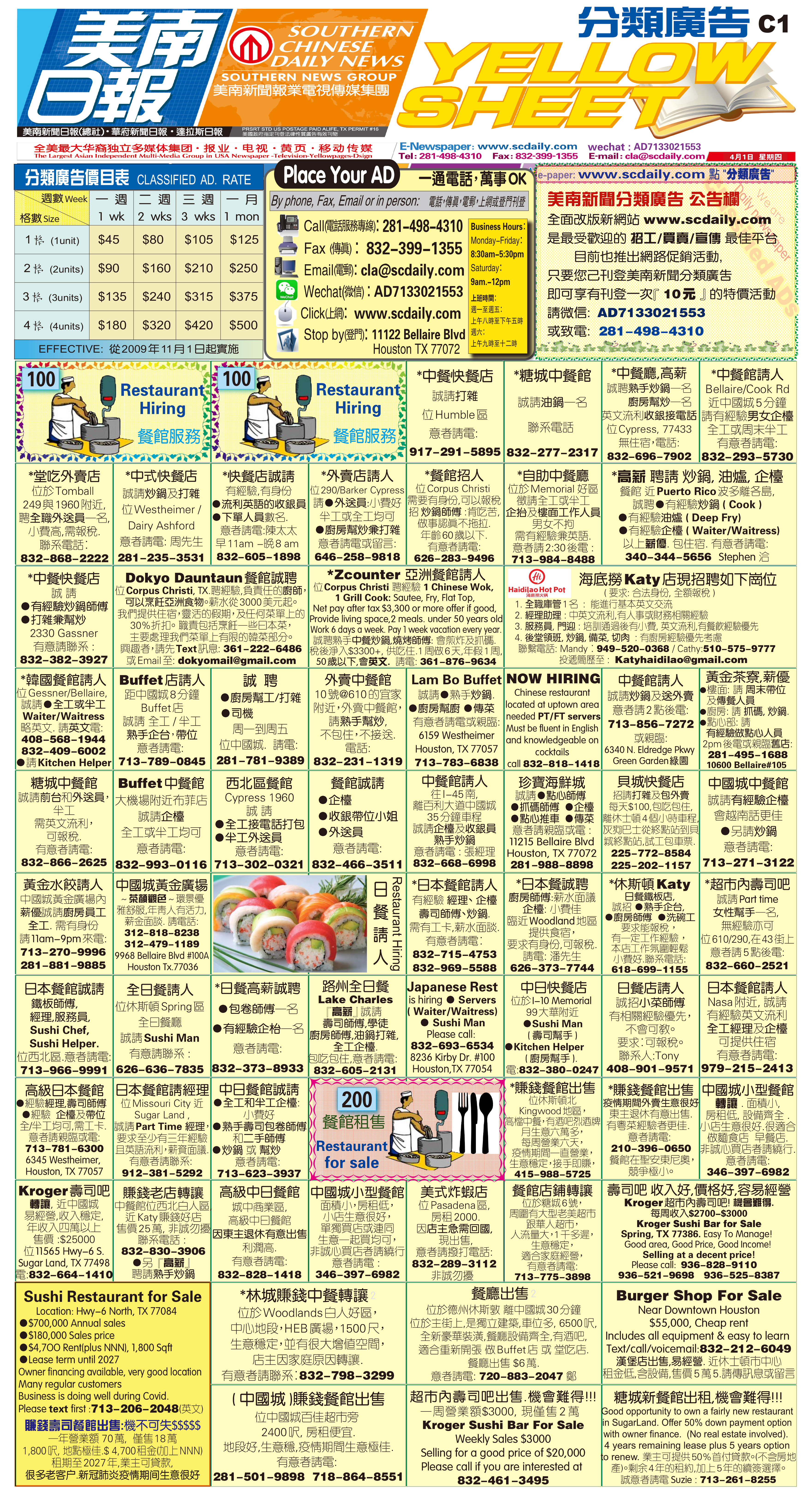210331 Epaper

 A Section B SectionC Section D Section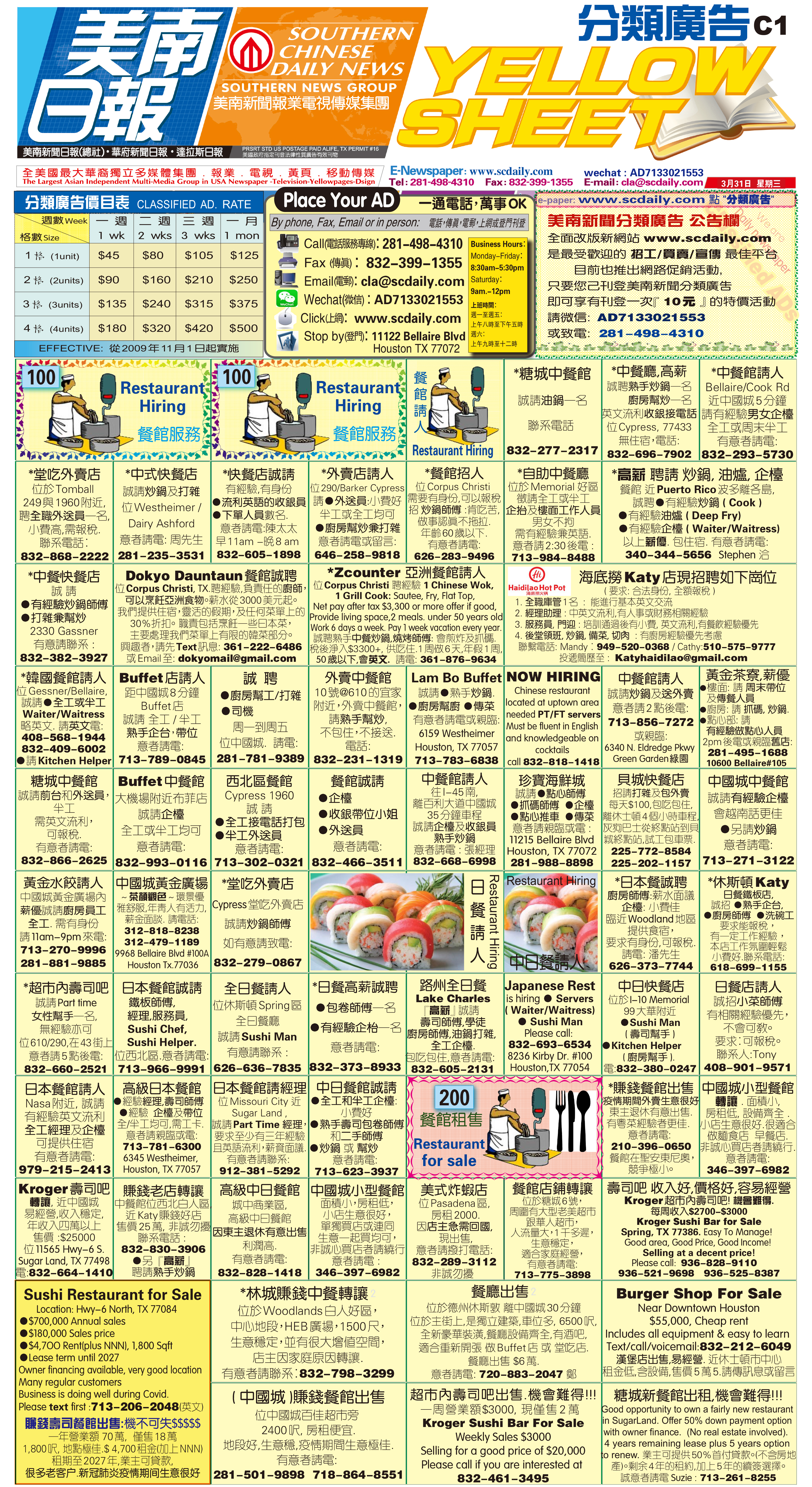210330 Epaper

 A Section B SectionC Section D Section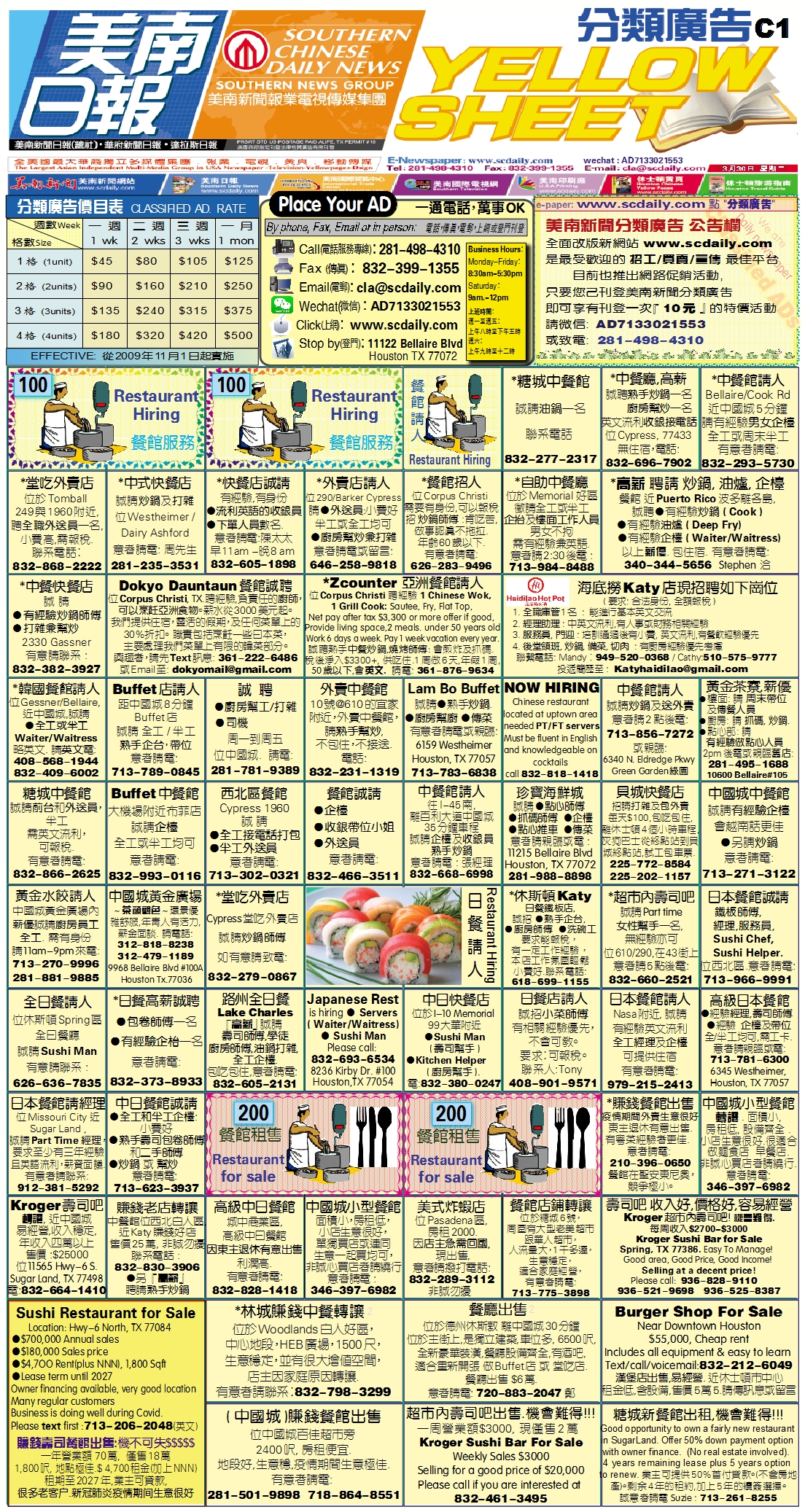210329 Epaper

 A Section B SectionC Section D Section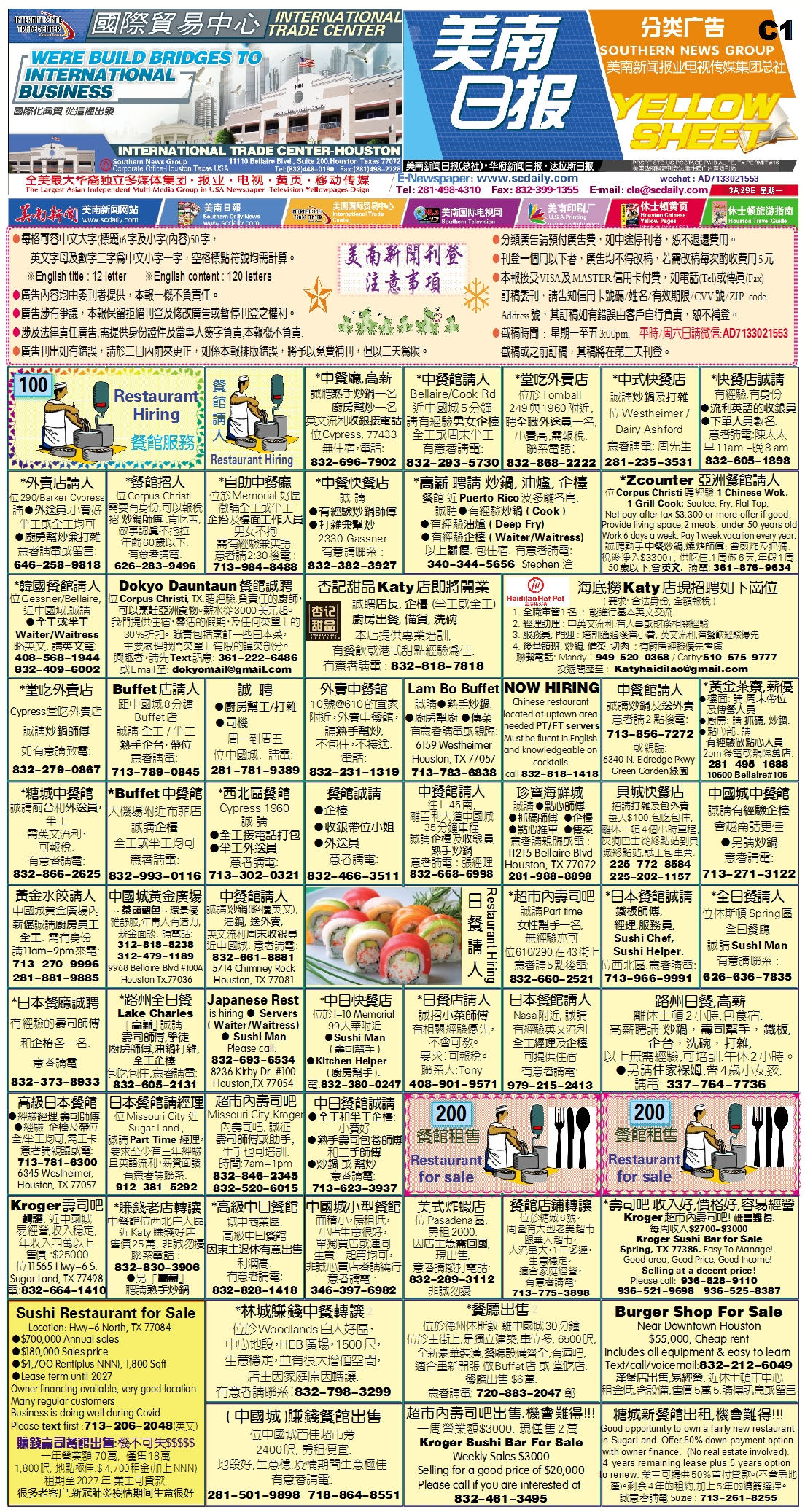210327 Epaper

 A Section B SectionC Section D Section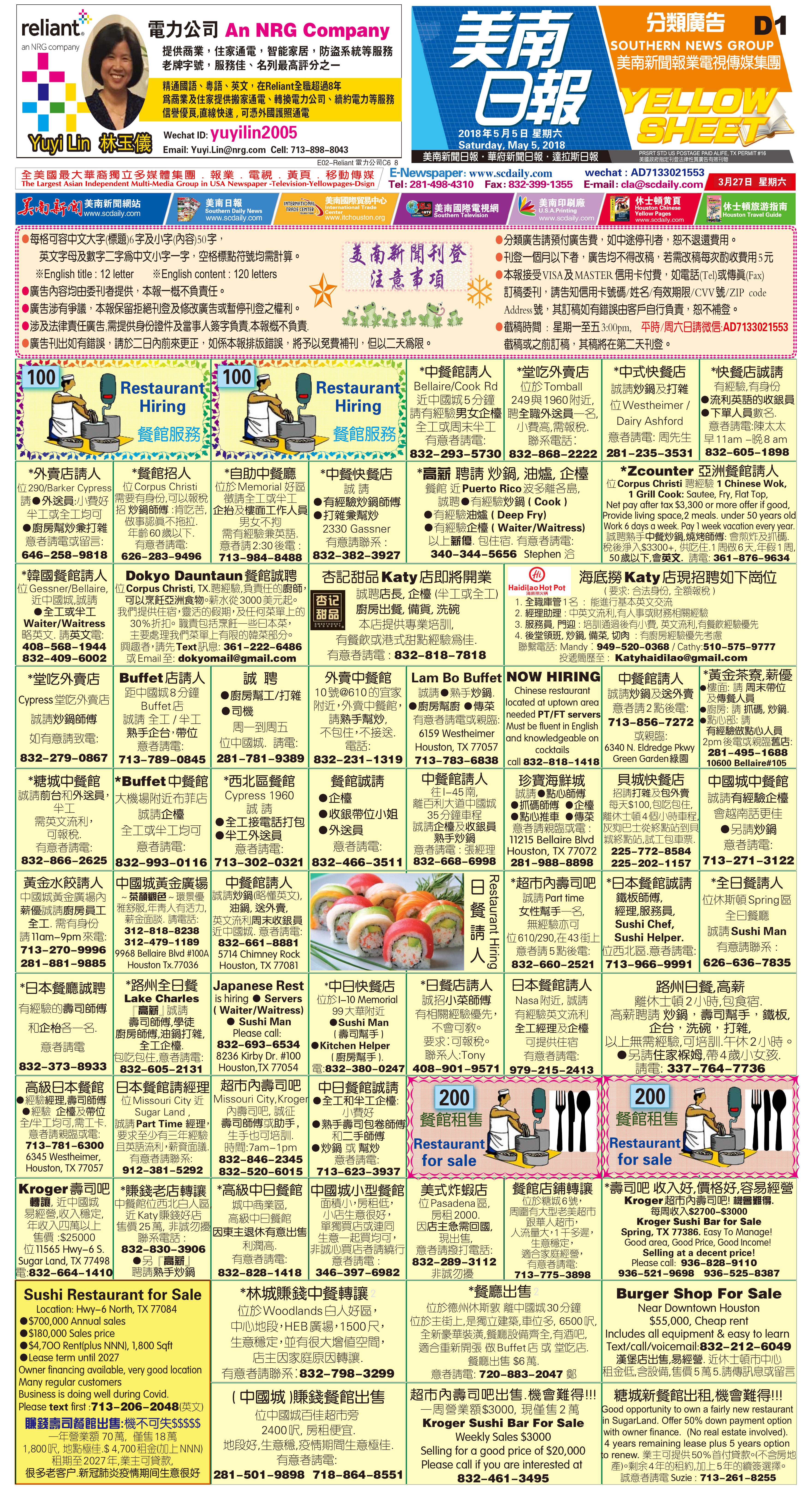210328 Epaper

 A Section B SectionC Section D Section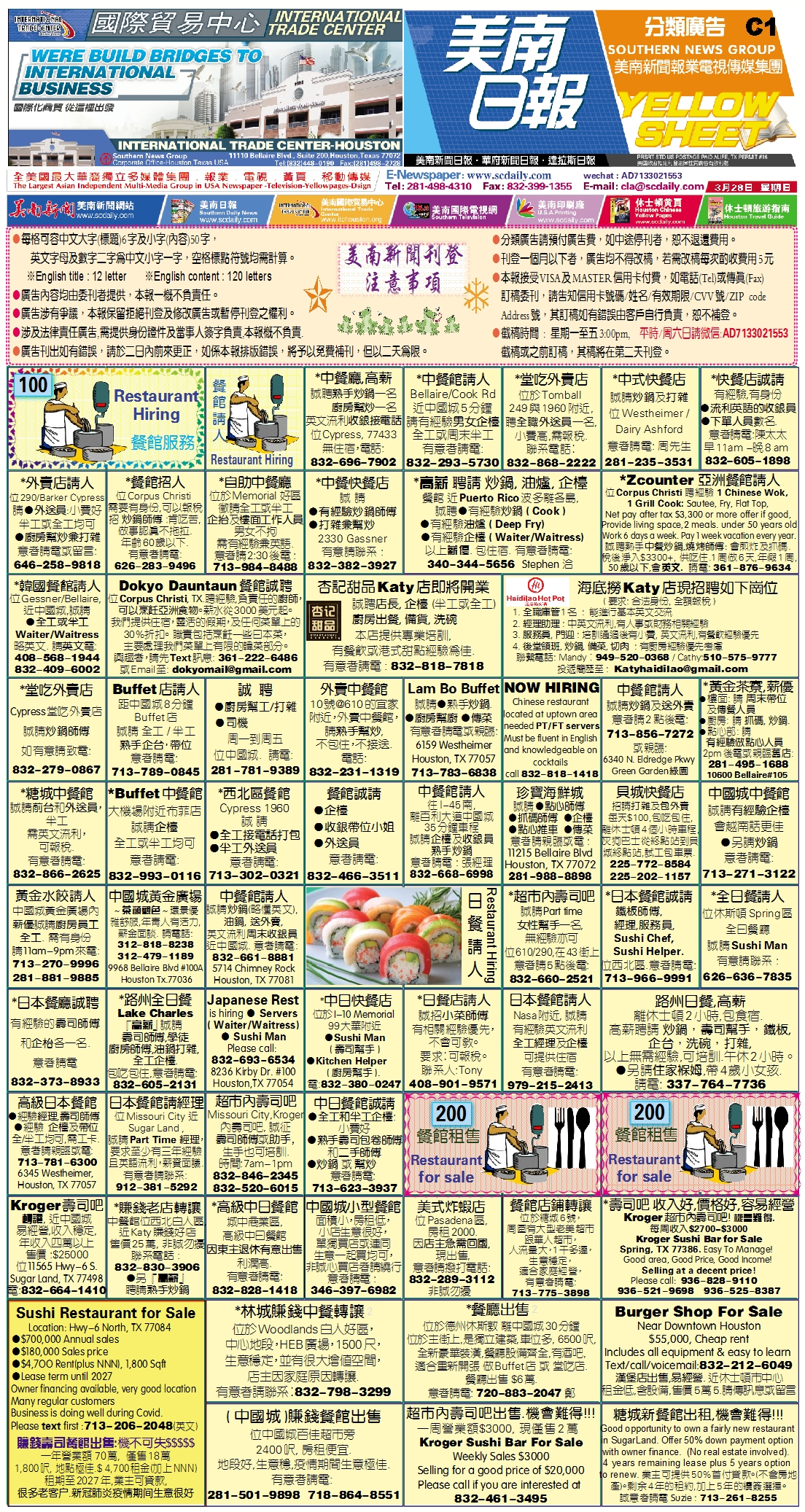210326 Epaper

 A Section B SectionC Section D Section